# For Loops

The for loop is perhaps the most commonly encountered loop in all programming. Its structure is the same in all programming languages, only its implementation is different. It is quite useful when you need to repeatedly execute a code segment, a certain number of times. The concept is simple. You tell the loop where to start, when to stop, and how much to increase its counter by each loop. The basic structure of a for loop is shown in Figure 5.2C.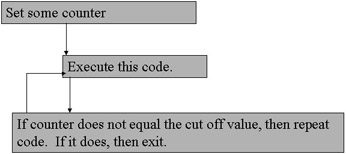Figure 5.2C: The structure of a for loop.

The essence of the for loop is in the counter variable. It is set to some initial value, it is incremented (or decremented) each time the loop executes. Either before or after each execution of the loop the counter is checked. If it reaches the cut-off value, stop running the loop.

The specific implementation of the for loop in C++ is shown here:

`for (int i = 0; i < 5; i++) { } `

What this code is doing is rather straightforward. First, we create an integer to be used as a loop counter (to count how many times the loop has executed) and we give it an initial value of zero. We then set the conditions under which the loop should execute. That’s the i < 5 portion of the code. It is essentially saying, “keep going as long as i is less than 5.”As soon as that condition is no longer true (i.e., i is equal to 5 or greater than 5), the loop stops executing. Finally, we have a statement to increment the loop counter after each iteration of the loop. Notice the semicolons in the for loop declaration. You should recall that all statements/expressions in C++ end with a semicolon. These code snippets are, indeed, valid statements and could be written as stand-alone expressions. The reason the last one does not have a semicolon is because it is followed by a closing parentheses and that terminates the expression. The three parts to the for loop declaration are the following.

1. Declare and initialize the loop counter.

2. Set the conditions under which the loop will execute.

3. Increment the loop counter.

### Example 5.4

Step 1: Use your favorite text editor to enter the following code.

`#include <iostream> using namespace std; int main() {  for (int j = 0; j < 10; j++)  { cout << "Going Up…." << j << "\n";  }// end of for loop  return 0; }// end of main`

Step 2: Compile the code.

Step 3: When you run the code, you will see something similar to what is displayed in Figure 5.3.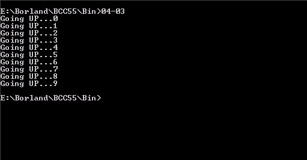Figure 5.3: For Loops.

This simple example will show you how a for loop works. Its rather simple but should give you the basic idea.

It should be pointed out that it is possible to increment by values greater than one, and even to count backwards, using the decrement operator. The next example illustrates this:

### Example 5.4a

Step 1:

`#include <iostream> using namespace std; int main() {  for (int j = 10; j >0; j—)  { cout << "count down… << j << "\n";  }// end of for loop  cout << "Blast Off!!! \n"; }// end of main`

Step 2: Compile the code.

Step 3: When you run this program you should see something like what is displayed in Figure 5.4A.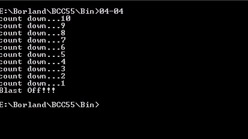Figure 5.4A: For loops 2.

You will find many programming situations where the for loop is quite useful, so it’s rather important. Also remember that for loops, like if statements, are found in all programming languages, only their implementation is different.

Loops, like if statements and switch statements, can be nested. You can have a loop inside of another loop. When you study algorithms in Chapter 13, and games in Chapter 14, you will see places where this is practically applied. The most important thing to remember when nesting loops is that the inner loop will execute a number of times equal to its counter multiplied by the outer loops counter. Put another way, if your outer loop executes 5 times, and you set the inner loop to loop 4 times, the inner loop will actually loop 20 times. It will loop 4 times, for each iteration of the outer loop. Let’s look at an example of this.

### Example 5.4b

Step 1: Enter the following code into a text editor and save it as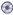05-04b.cpp.

`#include <iostream> using namespace std; int main() { for (int i = 0;i<3;i++) {   for(int j=0;j<4;j++)       { cout << "This is inner loop " <<  j ;      cout << " of outer loop " << i << endl;        }// end of inner loop  }// end of outer loop  return 0;    }// end of main`

Step 2: Compile and execute the code. You should see something like what is displayed in Figure 5.4B.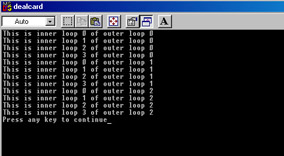Figure 5.4B: Nested for loops.

Note how often the inner loop printed out. This should prove to you the earlier statement regarding the number of iterations an outer loop makes. The outer loop, in this case, was set to loop 3 times. The inner loop was set to loop 4 times. Because the inner loop was nested within the outer loop, it actually looped 3 multiplied by 4, or 12 times.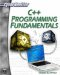C++ Programming Fundamentals (Cyberrookies)
ISBN: 1584502371
EAN: 2147483647
Year: 2005
Pages: 197
Authors: Chuck Easttom